# Texas Go Math Grade 2 Lesson 18.1 Answer Key Time to 15 Minutes

Refer to our Texas Go Math Grade 2 Answer Key Pdf to score good marks in the exams. Test yourself by practicing the problems from Texas Go Math Grade 2 Lesson 18.1 Answer Key Time to 15 Minutes.

## Texas Go Math Grade 2 Lesson 18.1 Answer Key Time to 15 Minutes

Essential Question
How do you tell time to 15 minutes on a clock?
Answer: The Quarter of the clock is 15 minutes on a clock

Explanation:
There can be two ways to say the time in the clock
It takes 5 minutes for the minute hand to move from one number to the next number on a clock face.
firstly, we can add on every minute of the clock up to they make a whole 15 minutes , or
secondly, adding up the 5 minutes three times can make a whole 15 minutes.

Explore
Draw the hour hand to show each time.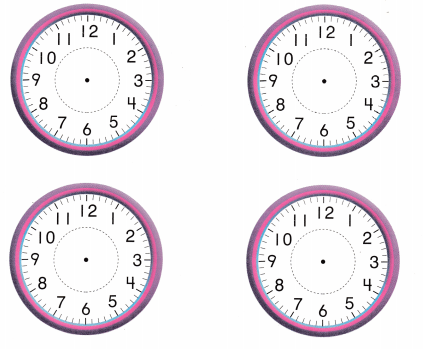For The Teacher

• Call out times to the hour and to the half hour Begin with 3:00. Have children draw the hour hand to show the time. Repeat the activity for hall past 5:00. 11:00, and half past 8:00.

Math Talk
Mathematical Processes
Describe where the hour hand points to show half past 4:00.
Answer: The hour hand points between 4 and 5 to show half past 4:00

Explanation: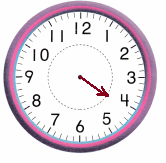To show half past 4:00 means the time is 4:30 so, making it as the half of an entire hour,
Then , an half of the hour is 30 minutes after the 4
So, The hour hand points between 4 and 5 to show half past 4:00

Model and Draw

It takes 5 minutes for the minute hand to move from one number to the next number on a clock face.The clock hands on these clocks show 4:15 and 4:30. These times are shown on the digital clocks.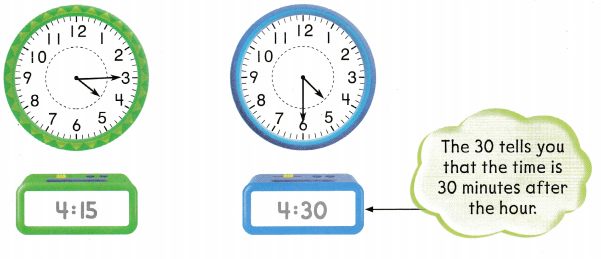Share and Show

Look at the clock hands. Write the time.

Question 1.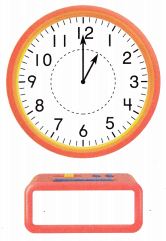Answer: The time on the clock is 1:00

Explanation:
The hour hand is on 1
and the minute hand is on 12, which makes a whole hour
Making it as 1:00 on the clockQuestion 2.Answer: The time on the clock is 8:30

Explanation:
The hour hand is between 8 and 9
and the minute hand is on 6, which makes 30 minutes on the clock
Making it as 8:30 on the clockQuestion 3.Answer:  The time on the clock is 3:45

Explanation:
The hour hand is between 3 and 4
and the minute hand is on 9 , which makes 45 minutes on the clock ,
for example , if the minute hand is on 6 , makes 30 minutes
but here it is on 9 that means add 15 minutes to the 30 minutes which results in 45 minutes
Making it as 3:45 on the clock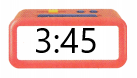Problem Solving

Look at the clock hands. Write the time.

Question 4.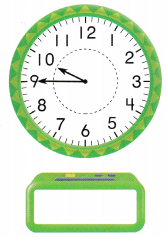Answer: The time on the clock is 9:45

Explanation:
The hour hand is between 9 and 10
and the minute hand is on 9 , which makes 45 minutes on the clock ,
for example , if the minute hand is on 6 , makes 30 minutes
but here it is on 9 that means add 15 minutes to the 30 minutes which results in 45 minutes
Making it as 9:45 on the clockQuestion 5.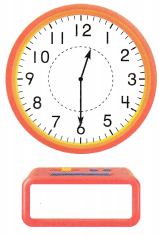Answer: The time on the clock is 12:30

Explanation:
The hour hand is between 12 and 1
and the minute hand is on 6, which makes 30 minutes on the clock
Making it as 12:30 on the clockQuestion 6.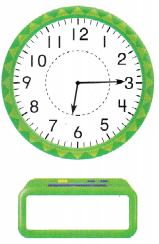Answer: The time on the clock is 6:15

Explanation:
The hour hand is between 6 and 7
and the minute hand is on 3, which makes 15 minutes on the clock
That is adding up the 5 minutes three times can make a whole 15 minutes.
Making it as 6:15 on the clock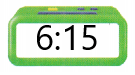Solve.

Question 7.
H.O.T. Multi-Step Allie eats lunch when the hour hand points between the 11 and the 12, and the minute hand points to the 3. Show this time on both clocks.
How do you know what time to write in the digital clock? Explain.Answer: Allie eats lunch at 11:15

Explanation:
Given, Allie eats lunch when the hour hand points between the 11 and the 12,
and the minute hand points to the 3.
Which means the time on the clock is 11:15The hour hand is between 11 and 12
and the minute hand is on 3, which makes 15 minutes on the clock
That is adding up the 5 minutes three times can make a whole 15 minutes.
Making it as 11:15 on the clock

Question 8.
H.O.T. Gabriel sees this time on a digital clock. Draw clock hands to show this time.Answer: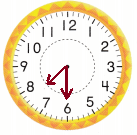Explanation:
The hour hand is between 7 and 8
and the minute hand is on 6, which makes 30 minutes on the clock
Making it as 7:30 on the clock

Question 9.
Mary goes to art class at 12:15. Which clock shows this time?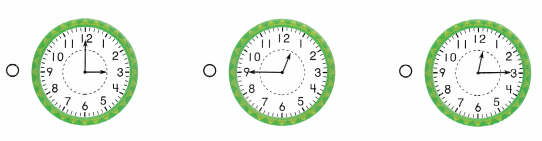Answer: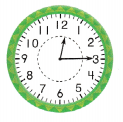Explanation:
The hour hand is between 12 and 1
and the minute hand is on 3, which makes 15 minutes on the clock
That is adding up the 5 minutes three times can make a whole 15 minutes.
Making it as 12:15 on the clock

Question 10.
Representations Which digital clock shows the same time as the clock to the right?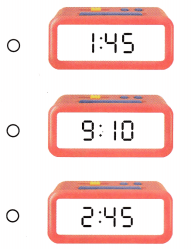Answer: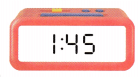Explanation:
The hour hand is between 1 and 2
and the minute hand is on 9 , which makes 45 minutes on the clock ,
for example , if the minute hand is on 6 , makes 30 minutes
but here it is on 9 that means add 15 minutes to the 30 minutes which results in 45 minutes
Making it as 1:45 on the clock

Question 11.
Texas Test Prep Reggie guitar lesson starts at 10:30. Which clock shows this time?Answer:Explanation:
Given, Reggie guitar lesson starts at 10:30.
The hour hand is between 10 and 11
and the minute hand is on 6, which makes 30 minutes on the clock
Making it as 10:30 on the clock.

Scroll to Top